## Screenshots

•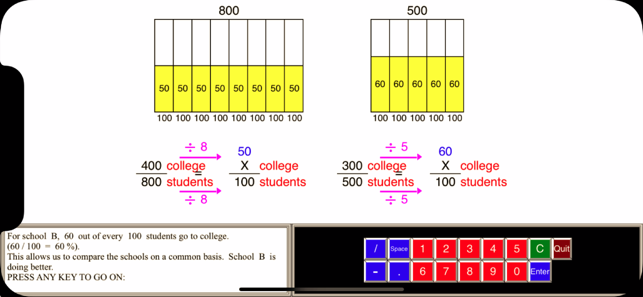•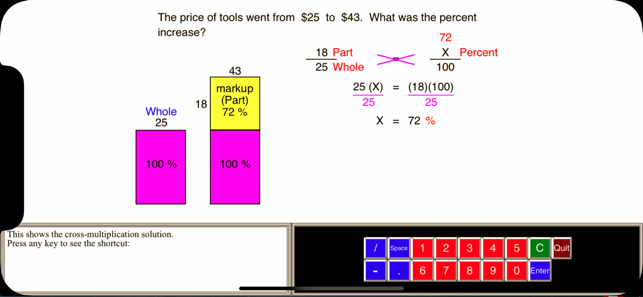••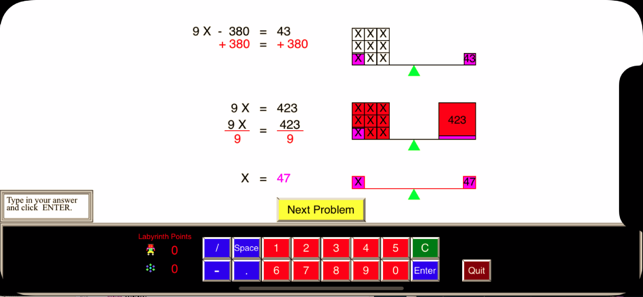•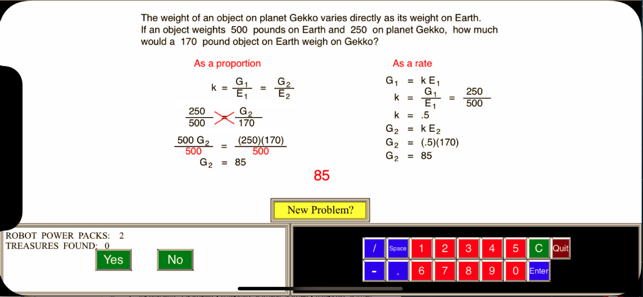•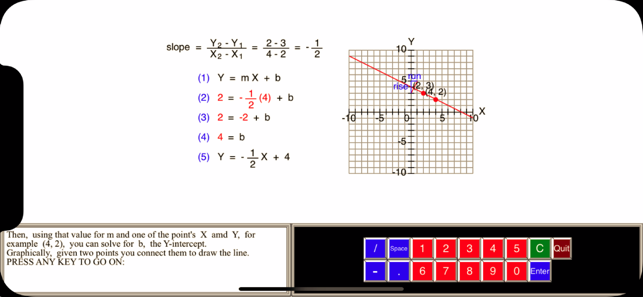•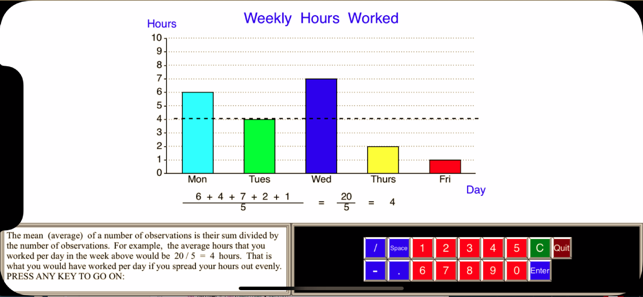•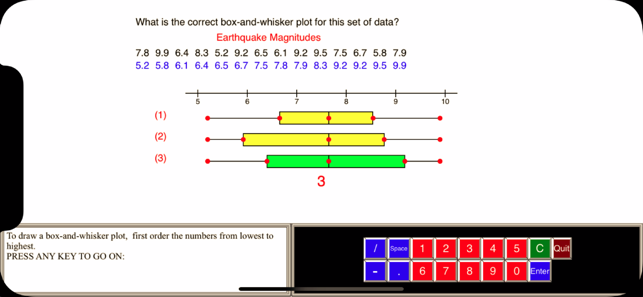•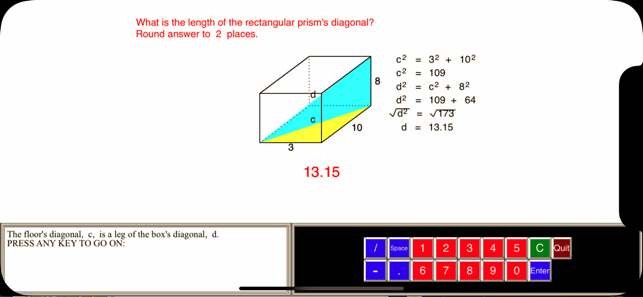•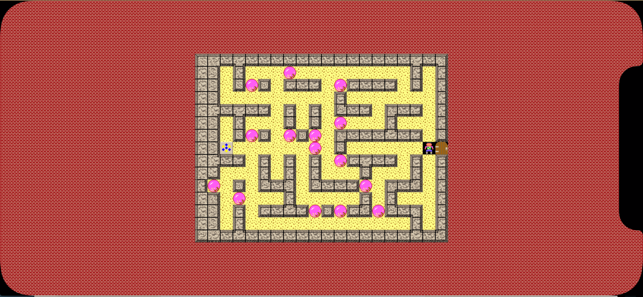## Description

Math Galaxy Pre-Algebra Fundamentals is like having an interactive textbook, with explanations, practice, games, and videos that cover all topics found in middle school math:

decimals
negative numbers (integers)
absolute value
powers/exponents
scientific and metric notation and operations
order of operations
factors and prime numbers
1 & 2-step equations
slopes and linear equations
ratios & proportions
similar figures
direct & inverse variation
percents
geometry, including length, area, volume, weight, lines, angles & polygons
pythagoras & right triangles
basic trigonometry
graphs
frequency tables,
mean (average) & median
stem-and-leaf plots
box-and-whisker plots
probability
patterns and sequences

The concepts are presented in bite-size pieces and there is an unlimited number of practice problems, with numbers chosen randomly.

By solving problems students earn robots to use in a maze game called the Labyrinth.

## What’s New

Version 2.1

Fixed repetition of problems in Ratios & Proportions exercise.

## Information

Seller
Gilbert Reilly
Size
95.5 MB
Category
Education
Compatibility

Requires iOS 8.0 or later. Compatible with iPhone, iPad, and iPod touch.

Languages

English

Age Rating
4+
Price
\$4.99

## Supports

•